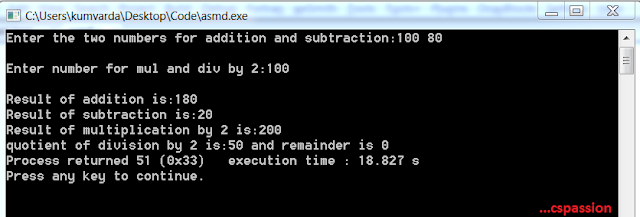## Addition,Subtraction,Multiplication by 2 and division by 2 without using +,-,* and / in c

On 04:16 by Vardan Kumar in    No comments

## Addition,Subtraction,Multiplication by 2 and division by 2 without using +,-,* and / in c

When it comes to enhance the software development skills, the magic lies in logic building. So here we are going to discuss a program with can add two numbers,subtract two numbers,multiply by 2 and division by 2,seems simple but here's a twist we can not use +,-,* and / operators for the same.

Now let us discuss the four functions one by one.Output

For the pupose of addition of two numbers off course not by using + operator, we will use ++(increment) as well as --(decrement) operator. Simple! isn't it?. And for the logic we will just decrement one of the number till it gets 0(in a loop) and increment the another one. Let us review the function definition for the same.

{
while(inum2--)
{
inum1++;
}
return inum1;
}

### 2. Subtraction:

Similarly for subtraction we'll use the combination of increment(++) as well as decrement(--) operators since we can not use '-' operator. And for the logic we'll just decrement onre of the number till it gets '0' and decrement the another one. Let us review function definition for the same.

int subtract(int inum1,int inum2)
{
while(inum2--)
{
--inum1;
}
return inum1;

}

### 3. Multiplication By 2:

For the purpose of multiplication by 2 we'll use already built addition function. Got the strike, yeah we will pass the number we want to multiply with '2' as arguments while calling "add" function and store the return value of add function in a pocket variable. Let us review the function definition for the same.

int mulby2(int inum)
{
int pocket;
return pocket;
}

### 4. Division By 2:

For the purpose of division by 2 we'll use bitwise operators, yes we got it right, the right shift operator(>>), we'll just right shift the number by '1' we want to divide with 2, hence we'll get the quotient for the division and if we want to get the remainder, off course we know we can use modulus operator(%)

int divby2(int inum)
{
inum = inum>>1;
return inum;
}

### Source Code:

#include<stdio.h>
{
while(inum2--)
{
inum1++;
}
return inum1;
}
int subtract(int inum1,int inum2)
{
while(inum2--)
{
--inum1;
}
return inum1;

}
int mulby2(int inum)
{
int pocket;
return pocket;
}
int divby2(int inum)
{
inum = inum>>1;
return inum;
}

void main()
{
printf("Enter the two numbers for addition and subtraction:");
scanf("%d %d",&num1,&num2);
printf("\nEnter number for mul and div by 2:");
scanf("%d",&num);
as=subtract(num1,num2);
am=mulby2(num);